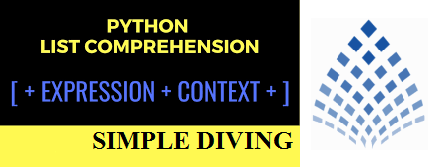Search

# Diving with List Comprehensions in Python

If you have used Python, may be you have used List Comprehensions also. You would have seen code like this -

numbers = [2,3,6,9] squares = [x ** 2 for x in numbers] squares #[4, 9, 36, 81]

This provides a List of squares of all the numbers passed as an input, this we know. There are some other interesting uses of List Comprehensions, lets have a look at them.Filtering or Applying Condition

A very interesting use of List comprehension is that it allows you to filter the data. Applying condition on List Comprehension reduces the no of lines of codes and also faster is the execution than other methods

Python has an easier way to solve this issue using List Comprehension. List comprehension is an elegant way to define and create lists based on existing lists.

Example 1) Finding odd numbers from a List of given numbers

odds = [x for x in range (1, 11) if x % 2 == 1] odds #[1, 3, 5, 7, 9]

We can extend above example to find some power or any other complex pattern for that matter, using List Comprehensions

Example 2) Finding squares of the odds numbers

odd_squares = [x ** 2 for x in range (1, 11) if x % 2 == 1] odd_squares #[1, 9, 25, 49, 81]

Example 3) Find out the palindromes from a List of the words

words = ['level','maths','stats'] palindromes = [word for word in words if word==word[::-1]] palindromes #['level', 'stats']

Example 4) Suppose we need to read a file and store it text in List of lists by storing each line in a list and storing all comma separated values as an element of that list

data = [line.strip().split(",") for line in open("data.txt") ] data

Python supports other types of objects with the same Comprehension technique. Comprehensions aren’t just for lists.

Dictionary Comprehension

Example 5) We can use same List Comprehension technique, even to create a Dictionary object

numbers = [2,4,6,8,14] dict_squares = {number : number ** 2 for number in numbers} dict_squares #{2: 4, 4: 16, 6: 36, 8: 64, 14: 196}

The above example allow to create a Dictionary object containing number : square as its

key : value pattern

We have covered some very simple but useful methods of using List Comprehensions. Try practicing them in your coding, it will make an impact both in faster execution while having less no. of lines in code.

Points to Ponder

• List comprehension is an elegant way to define and create lists by transforming existing lists

• List comprehension is generally more compact and faster than normal functions and loops for the same task

• In order to keep user-readability, avoid writing very long list comprehensions in one line

• Every list comprehension can be rewritten in for loop, but vice-versa is not always true